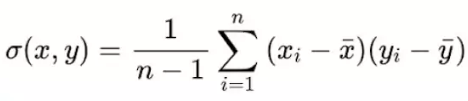top of page
Search

# Unraveling the Relationship: Covariance and Correlation in Statistical Analysis

Introduction:In today’s big data world, we deal with a wide range of variables to perform various data analytics operations. Finding relationships between variables helps us to deduce meaningful insights that can help organizations make better-informed decisions. For instance, is there a relationship between coronavirus strength and the immune status of a person? Can you interpret this? Similarly, Is there a relationship between tax rates and economic growth of a state? How can you explain this? These examples of scrutinizing the relationship between variables can be quantified by employing statistical analysis tools such as covariance and correlation

What is Covariance?

Covariance is a statistical measure that describes the relationship between two random variables. It measures how changes in one variable are associated with changes in another variable. In other words, covariance indicates the direction of the linear relationship between two variables and whether they tend to vary together.

Mathematically, the covariance between two variables, let's say X and Y, is denoted as Cov(X, Y) or σ(X, Y). It is calculated as the average of the products of the deviations from the mean for each variable. The formula for covariance is:• xi represents the values of the X-variable

• yi represents the values of the Y-variable

• x represents the mean (average) of the X-variable

• y represents the mean (average) of the Y-variable

• n represents the number of data points

Different types of Covariance:

• Positive Covariance: Positive covariance indicates that when one variable increases, the other variable tends to increase as well. Similarly, when one variable decreases, the other variable tends to decrease. It suggests a positive linear relationship between the variables.

• Negative Covariance: Negative covariance indicates that when one variable increases, the other variable tends to decrease, and vice versa. It suggests a negative linear relationship between the variables.

• Zero Covariance: Zero covariance indicates no linear relationship between the variables. It means that changes in one variable are not associated with changes in the other variable.What is Correlation?

Correlation is a statistical measure that quantifies the strength and direction of the linear relationship between two variables. It measures the degree to which changes in one variable are associated with changes in another variable.

The correlation coefficient is calculated by dividing the covariance between the two variables by the product of their standard deviations. The formula for calculating the correlation coefficient (r) is:σ(X) and σ(Y) are standard deviations with respect to X and Y.

The correlation coefficient, denoted by the symbol "r," is used to represent correlation. The correlation coefficient ranges between -1 and 1, where:

• A correlation coefficient of 1 indicates a perfect positive linear relationship. It means that as one variable increases, the other variable also increases in a linear fashion.

• A correlation coefficient of -1 indicates a perfect negative linear relationship. It means that as one variable increases, the other variable decreases in a linear fashion.

• A correlation coefficient of 0 indicates no linear relationship between the variables. It means that changes in one variable are not associated with changes in the other variable.Different types of Correlation:

Pearson Correlation: Pearson correlation, also known as the Pearson product-moment correlation coefficient, is a measure of the linear relationship between two continuous variables. It assesses the strength and direction of the linear association. Pearson correlation assumes that the relationship between variables is linear and that the variables are normally distributed.

Spearman Correlation: Spearman correlation is a non-parametric measure of correlation that assesses the monotonic relationship between variables. It is used when the variables are not necessarily linearly related, but there is a consistent monotonic association between them. Spearman correlation is based on the ranks of the variables rather than their actual values.

Implementation of Covariance and Correlation in R:

Step 1: Create a vector or variables to represent your data. Let's say we have two variables, X and Y, and we have stored them in separate vectors.

```X <- c(1, 2, 3, 4, 5)
Y <- c(2, 4, 6, 8, 10)

```

Step 2: Use the cov() and cor() function to calculate the covariance and correlation between X and Y and print it.

```covariance <- cov(X, Y)
correlation <- cor(X, Y)

print(covariance)
print(correlation)```

Implementation of Covariance and Correlation in python:

Step 1: Import the NumPy library.

```import numpy as np

```

Step 2: Create two NumPy arrays or lists to represent your variables, let's say X and Y.

```X = np.array([1, 2, 3, 4, 5])
Y = np.array([2, 4, 6, 8, 10])

```

Step 3: Use the np.cov() and np.corrcoef() function to calculate the covariance and correlation between X and Y and print it.

```covariance = np.cov(X, Y)[0, 1]
correlation_matrix = np.corrcoef(X, Y)[0, 1]

print(covariance)
print(correlation)

```

The [0, 1] indexing is used to extract the covariance value from the covariance matrix. The covariance matrix returned by np.cov() provides the covariances between all pairs of variables.

Differences b/w Correlation and Covariance:

Covariance:

1. Covariance is scale-dependent.

2. Covariance can take any real value.

3. The strength of covariance is difficult to interpret directly as it depends on the scales of the variables.

Correlation:

1. Correlation is scale-independent

2. Correlation ranges between -1 and 1

3. The magnitude of the correlation coefficient directly indicates the strength of the linear relationship between variables. A correlation coefficient close to -1 or 1 indicates a strong linear relationship, while a coefficient close to 0 suggests a weak or no linear relationship.